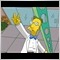3 equal conditions, which type of loop?1169

so if you have an indicator that is made of other (iCustom) indi's signals,

A,B & C, which are boolean (only 1/0 values). And I want this for result, which type of loop should I use

If

none of the 3 (A,B,C) is =1 and all 3 are = 0 then result is 0

1 of the 3 (A or B or C) is =1 and the other 2 are =0 then result is 0

2 of the 3 (A&B or B&C or A&C) is =1 and the other 2 are =0 then result is 1

all the 3 (A,B,C) are =1 so none of the 3 =0 then result is 1

so 0 if maximally 1 is 1, 1 if 2 or more are 1..504

if (A + B + C) < 2

Result=0;

else

Result=1;

That is, if your third line was mistyped.1169

blogzr3:

if (A + B + C) < 2

Result=0;

else

Result=1;

That is, if your third line was mistyped.

it was mistyped, thx; the answer looks kind of obvious now...